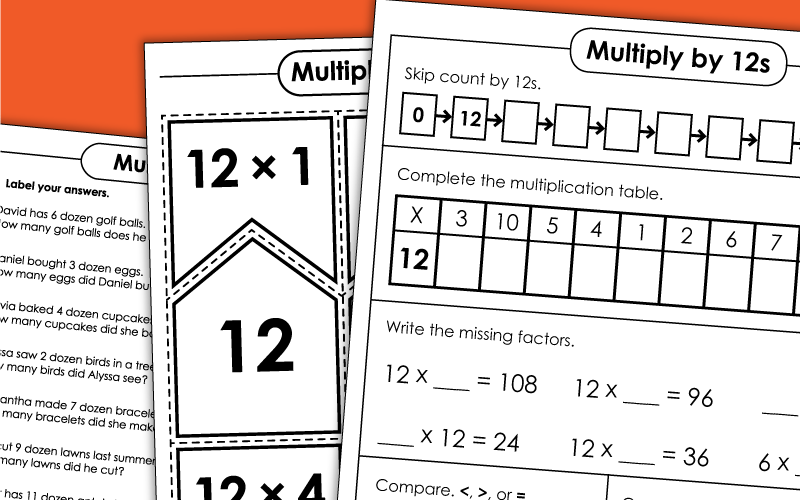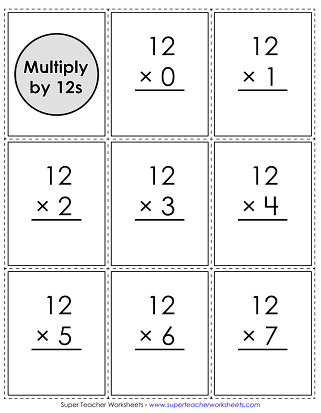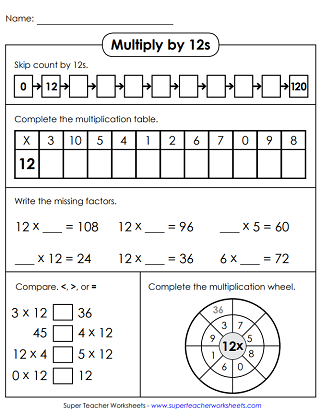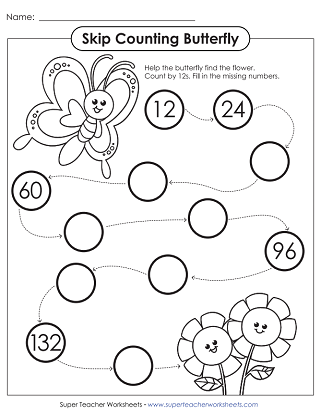# Multiplication by 12s

With the printable worksheets on this page, students will practice multiplying by 12s.

If you're looking for worksheets with all facts from 0-12, please jump to our Basic Multiplication 0-12 page.## Multiplication by12s Only

Print this worksheet for your class so they can learn to multiply by 12s. Find the missing factors, complete the multiplication wheel, and skip counting by 12s are just a few of the activities on this worksheet.
Multiply by 12s in each circle to solve the math problems.
Printable word problem worksheet. Each problem requires students to multiply by dozens. example: Olivia baked 4 dozen cupcakes. How many cupcakes did she bake?
There is an array of different activities to be played with these printable puzzle pieces. These multiplication facts feature the numbers 11 and 12.
Print this origami toy. Cut, color, and fold to make a toy/game that helps kids learn how to multiply by 12s.
Color and cut out the dog. THen cut out the multiplication fact strip. Slide it through the dog to reveal multiplication facts (with or without answers). Use this tool to practice multiplication facts that have 12 as a factor.
This file has 11 flash cards, 5 super challenge flash cards, a self-test, and a mat for sorting.

## Skip CountBy 12s

Answer 8 math questions to demonstrate an understanding of skip counting by 12s.
Help the butterfly find the flower by skip counting.
Skip count by 12s. Write the missing numbers on the penguin bellies.

## All Facts0s through 12s

All Multiplication Facts 0-12

Multiplication Facts 0-10

This page covers all basic multiplication facts with factors between 0 and 10.

More Basic Multiplication

Navigate to other sections of Super Teacher Worksheets with basic multiplication worksheets. We have pages with resources on arrays, pages with fact family resources, and much, much more.

## Sample Worksheet ImagesMy Account
Site Information